# Class 10 Science Numerical Questions for Electricity

Given below are the Class 10 Science Solved Numerical Questions for electricity

Question 1
A wire of length 3 m and area of cross-section 1.7 × 10-6 m2 has a resistance 3 × 10-2 ohm.
a. What is the formula for resistivity of the wire and what is the unit of it
b. Calculate the resistivity of the wire
Solution
a. Resistivity of the wire is given by
$\rho =R\frac{A}{L}$
And It unit is Ohm-m
b. In this case
L=3 m
$A=1.7 \times 10^{-6}$ m2
$R=3 \times 10^{-2}$ ohm
So
$\rho= 1.7 \times 10^{-8}$ Ohm-m

Question 2
The table given below shows the resistivity of three Material X, Y and Z?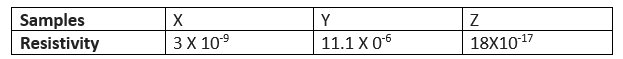a. Arrange the samples in increasing order of conductivity
b. Which of these is best conductor?
c. Which are these is best insulator?
Solution
a.Conductivity is inversely proportional to resistivity
So
Y< X< Z
b. Z is the best conductor as it has least resistivity
c. Y is the best insulator as it has highest resistivity

Question 3.
There are m resistor each of resistance R. First they all are connected in series and equivalent resistance is X. Now they are connected in parallel and equivalent resistance is Y.  What is the ratio of X and Y?
Solution
Series combination
$X=R+R+R+......m \; times = mR$
Parallel combination
$\frac{1}{Y}=\frac{1}{R}+\frac{1}{R}+\frac{1}{R}+...=\frac{m}{R}$
Or $Y=\frac {R}{m}$
So X : Y=m2 : 1

Question 4
We have four resistors A ,B ,C and D of resistance 4 ohm,8 ohm ,12 ohm and 24 ohm respectively?
(i)Lowest resistance which can be obtained by combining these four resistors
(ii)Highest resistance which can be obtained by combining these four resistors
Solution
Lowest resistance is obtained in parallel combination
$\frac{1}{R}=\frac{1}{4}+\frac{1}{8}+\frac{1}{12}+\frac{1}{24}$
Or R=2Ω
Highest resistance is obtained in series combination
$R=4+8+12+24=48\Omega$

Question 5
Three resistors  5Ω, 10Ω and 30Ω are connected in parallel with the battery of Voltage  6V?
(i)The value of current across each resistor
(ii)The value of Potential difference across each resistor
(iii)Total current in the circuit
(iv)Effective resistance of the circuit
Solution
Potential difference remains same across parallel combination
So current in each resistor is calculated as
I1=V/R1=6/5=1.2 A
I2=V/R2=6/10=.6 A
I3=V/R3=6/30=.2 A
Total current in the circuit
I=I1+I2+I3=1.2+.6+.2=2 A
Effective resistance
$\frac{1}{R}=\frac{1}{5}+\frac{1}{10}+\frac{1}{30}$
R= 3Ω

Question 6
An electric bulb draws a current of .8 A and works on 250 V on the average 8 hours a day.
a. Find the power consumed by the bulb
b. If the electric distribution company changes Rs 5 for 6 KWH, what is the monthly bill for 60 days
Solution Power of the electrical bulb is given by
$P=V \times I=.8 \times 250 = 200 W =.2 KW$
Total energy consumption by the bulb in 60 days
$E=P \times t= .2 \times 8 \times 60=96 KWH$
So, cost will be = $\frac {5 \times 96}{6}=Rs 80$

Question 7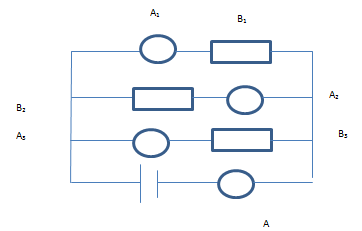A1 ,A2 ,A3 and  A are ammeters connected in the circuit
B1 ,B2 and B3 are three identical bulbs
They all are connected to Voltage source as shown in Figure
When the three bulb are working good and glowing ,the current recorded in Ammeter A is 6 A
a. Same amount of current will go through each Bulb.  And the value is 2 A .True or False
b. If the Bulb B3is blown away, the bulb B1 and B2 will start glowing more. True or False
c. What will happen to all the ammeter reading if Bulb B1 is blown away
d. The current shown in Ammeter A remains  even any bulb goes down. True or False
Solution
a. Since Bulb are identical and connected in parallel with Voltage. Same current will flow through each bulb. Since the total current is 6 A.Individual current will be 2 A
b. If the Bulb B3 is blown away, The potential difference across other bulb still remains same, So same current will flow and they will glow as it is. No change
c. when Bulb B1 goes down, the current in that part become zero.
So reading of Ammeter A1 becomes zero
Reading of Ammeter A2 will remain same i.e. 2 A
Reading of Ammeter A3 will remain same i.e. 2 A
Reading of Ammeter A will be = 2+2=4 A
d. As shown above, the reading of Ammeter A will change

Question 8
Give the formula for each
(i)Ohm’s Law
(ii)Resistance in terms of Length,Area,resistivity
(iii)Current in terms of Resistance and Voltage
(iv)Equivalent Resistance for Resistors in Series
(v)Equivalent Resistance for Resistors in Parallel
(vi)Power produced in the resistance
Solution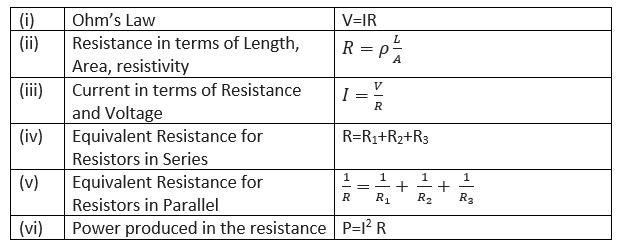Question 9
Two students (P) and (Q) connect their two given resistors $R_1$ and $R_2$ in the manners shown below :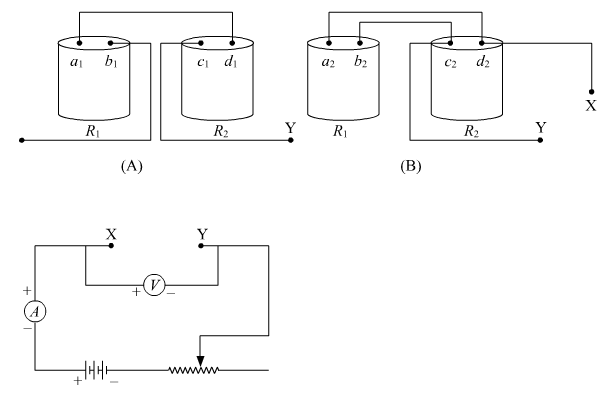Student (P) connects the terminals marked ($b_1$) and ($c_1$) while student (Q) connects the terminals marked ($d_2$) and ($c_2$) in their respective circuits at the points marked X and Y.
Which one of the following is correct in relation to the above arrangements?
a. both the students will determine the equivalent resistance of the series combination of the two resistors.
b. both the students will determine the equivalent resistance of the parallel combination of the two resistors
c. student (P) will determine the equivalent resistance of the series combination while student (Q) will determine the equivalent resistance of the parallel combination of the two resistors.
d. student (P) will determine the equivalent resistance of the parallel combination while student (Q) will determine the equivalent resistance of the series combination of the two resistors.
Solution
In combination made by student A, only one end of the resistors are in contact so they are connected in series. But, in the combination made by student B, both the ends of both the resistances are connected together, so they are connected in parallel.
So, Student P will measure the equivalent resistance for series combination and Student Q will measure for parallel combination
Hence, the correct answer is option c.

Question 10
which one of the below circuit is properly connected with the electrical components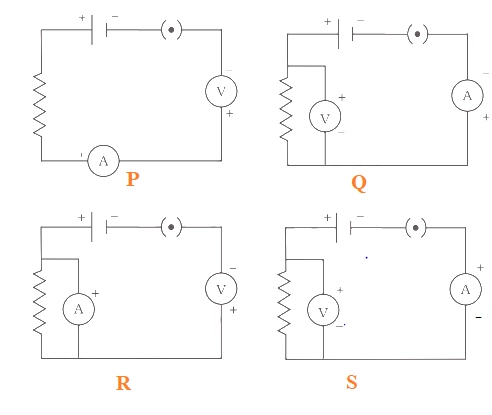a. P
b. Q
c. R
d. S
Solution
Ammeter should be in series and Voltmeter in parallel. Also current flows from + to -. So (b) is the correct option

Question 11
Judge the equivalent resistance when the following are connected in parallel
(a) 1 Ω and $10^6$ Ω
(b) 1 Ω, $10^3$ Ω and $10^6$ Ω.
Solution

(a) $\frac{1}{R}=\ \frac{1}{1}+\ \frac{1}{10^6\ }=\ \frac{10^6+1}{10^6}$
$= \frac{1000000+1}{1000000}=\ \frac{1000001}{1000000}$
$= \frac{1000000}{1000001}=0.9 \Omega$
(b) $\frac{1}{R}=\ \frac{1}{1}+\ \frac{1}{10^3}+\ \frac{1}{10^6}=\ \frac{10^6+10^3+1}{10^6}$
$= \frac{1001001}{1000000}$
$R = \frac{1000000}{1001001}=\ 0.9 \Omega$

Question 12
How can three resistors of resistances 2 Ω, 3 Ω and 6Ω be connected to give a total resistance of (a) 4 Ω, (b) 1 Ω?
Solution
(a) for 4 Ω reistance:
3Ω and 6 Ω resistances are connected in parallel and this combination is connected in series to 2 Ω resistor.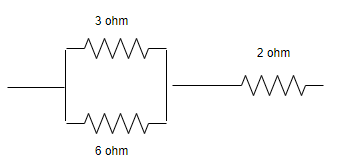(b) For 1 Ω resistance:
All these three resistances are connected in parallel to get equivalent resistance of 1 Ω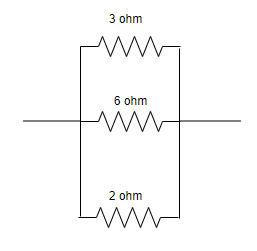Question 13
What is (a) the highest, (b) the lowest total resistance that can be secured by combinations of four coils of resistances 4 Ω, 8 Ω, 12 Ω, 24 Ω?
Solution
(a) For the highest resistance, resistors are connected in series:
$R = R_1 + R_2 + R_3 + R_4$
= 4 + 8 + 12 + 24
R = 48 Ω
(b) The lowest resistance will be obtained when these are connected in parallel:
$\frac{1}{R}=\ \frac{1}{R_1}+\ \frac{1}{R_2}+\ \frac{1}{R_3}+\ \frac{1}{R_4}$
$\frac{1}{R}=\ \frac{1}{4}+\ \frac{1}{8}+\ \frac{1}{12}+\ \frac{1}{24}$
$\frac{1}{R}=\ \frac{6+3+2+1}{24}=\ \frac{12}{24}$
$\frac{1\ }{R}=\ \frac{1}{2}$
R = 2 Ω

Question 14
An electric motor takes 5 A from a 220 V line. Determine the power of the motor and the energy consumed in 2 h.
Solution
Power=VI
$P= 220 \times 5$
P= 1100 Watts
$\text {Energy} = \text {Power} \times \text{Time}$
$= 1100 \times 2 \times\ 3600$ sec
$= 7,920,000 = 7.92 \times\ 10^6$ joules
$= 7.92\times\ 10^3$ kilojoules

Question 15
An electric lamp of 100 Ω, a toaster of resistance 50 Ω, and a water filter of resistance 500 Ω are connected in parallel to a 220 V source. What is the resistance of an electric iron connected to the same source that takes as must current as all three appliances and what is the current through it?
Solution
The electric iron will have equivalent resistance to the same as 100 Ω, 50 Ω and 500 Ω resistors are in parallel.
Let it be R Ω.
Therefore
$\frac{1}{R}=\ \frac{1}{100}+\ \frac{1}{50}+\ \frac{1}{500}$
$= \frac{5+10+1}{500}=\ \frac{16}{500}$
$R = \frac{500}{16}\ =31.25$ Ω
Also Current is given as
$I = \frac{V}{R}=\ \frac {220}{31.25}$
I = 7.04 A
Question 16.
An electric iron consumer's energy at a rate of 880 W when heating is at the maximum rate and 360 W when the heating is at the minimum,the voltage is 220 V. What are the current and the resistance in each case ?
Solution
Case A : Maximum rate
P = 840W
V = 220V
We know
$P = VI$
or
$I = \frac {P}{V} = \frac {840}{220}$
I = 4 A
Now
$R = \frac {V}{I} = \frac {220}{4} = 55$ Ω

Case B: Minimum rate
P = 360W
V = 220V
Again
P = VI
or
$I = \frac {P}{V} = \frac {360}{220}$
I = 1.636 A
Now
$R = \frac {V}{I} = \frac {220}{1.636} = 134.45$ Ω
Question 17.
A student has a resistance wire of 1 ohm. If the length of this wire is 50 cm, to what length he should stretch it uniformly so as to obtain a wire of 4 Ω resistance? Justify your answer.
Solution
Let $\rho =a$
$R=\rho \frac {L}{A}$
$1 = a \frac {50}{A}$
or A= 50a

Now as Volume remains constant
$A_1L_1 = A_2 L_2$
$2500a= A_2 L_2$
Now
$R=\rho \frac {L}{A}$

$4 = a \frac {L}{A}$
$4 =a \frac {L}{2500a/L}$
$L^2 = 4 \times 2500$
$L^2 = 10000$
L=100 cm
So lenght should be doubled

Question 18.
Calculate the resistivity of the material of a wire of length 1 m, radius 0.01 cm and of resistance 20 ohms.
Solution
$R=\rho \frac {L}{A}$
$r = 0.01 cm = 1 \times 10^{-4}$ m
$A = \pi r^2= 3.14 \times (1 \times 10^{-4} )^2 = 3.14 \times 10^{-8}$ m2

Now, $\rho = \frac {RA}{L}$
$=\frac {20 \times 3.14 \times 10^{-8} }{1}$
$=6.28 \times 10^{-7}$ ohm-m

Question 19.
There are three resistors of 10 , 20 and 30 joined in parallel in a circuit. The potential difference across the electric circuit is 10 V.
(a) Draw a circuit diagram for the above case.
(b) Find the total resistance of the combination of resistors.
(c) Calculate the electric current drawn from the same source.

Question 20.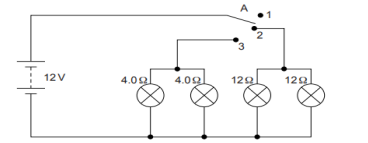(i) State what happens when switch A is connected to
(a) Position 2
(b) Position 3
(ii) Find the potential difference across each lamp when lit.
(iii) Calculate the current
(a) in each 12 ohm lamp when lit.
(b) In each 4ohm lamp when lit.
(iv) Show, with calculations, which type of lamp, 4.0 ohm or 12 ohm , has the higher power.
Solution
(i)
(a) 12 ohm lamps (only) on.
(b) 4 ohm lamps (only) on
(ii) 12 V for both sets of lamps and all of them are in parallel.
(iii)
(a) 12 ohm lamps are on when the wire is connected to position 2.
Voltage across both 12 ohm lamps = 12 V.
V=IR (Ohm’s law).
I=V/R= 12/12=1 A
(b) 4 ohm lamps are on when the wire is connected to position 3.
Voltage across both 4 ohm lamps = 12 V.
V=IR (Ohm’s law).
I=V/R= 12/4=3 A
(iv)All lamps are in parallel and hence same V for all lamps.
For 4 ohm lamps $𝑃 = \frac {V^2}{R}= 36W$
For 12 Ω lamps $𝑃 = \frac {V^2}{R}= 12W$
Hence 4 ohm lamps will have higher power.

Question 21.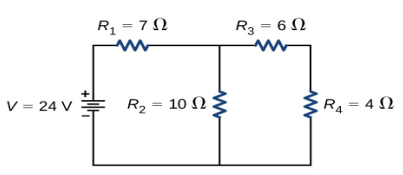(i)Calculate the total resistance of the circuit
(ii)find the total current in the circuit.
Solution
(i)Resistance R3 and R4 so, equivalent R'= 6 + 4 = 10 ohm
Now R and R2 are in parallel, equivalent resistance R" is
$\frac {1}{R"}= \frac {1}{10} + \frac {1}{10}$
R"=5 ohm
Now R" and R1 are in series, So equilvalent resistance of the whole circuit is
R=5 + 7=12 ohm
(ii) I= V/R= 24/12=2A